Courses

# Extra Questions and Practice Exercise- Data Handling Class 8 Notes | EduRev

## Class 8 : Extra Questions and Practice Exercise- Data Handling Class 8 Notes | EduRev

The document Extra Questions and Practice Exercise- Data Handling Class 8 Notes | EduRev is a part of the Class 8 Course Mathematics (Maths) Class 8.
All you need of Class 8 at this link: Class 8

Question 1: Given below are the marks obtained by 30 students of a class in a test.

10, 8, 12, 17, 19, 17, 41, 40, 12, 46, 37, 17, 1, 9, 21, 13, 48, 12, 21, 19, 33, 5, 38, 39, 19, 23,2, 6, 30, 27.

Using tally marks prepare a frequency table such that 20–30 is a class interval.

Solution:

The lowest observation = 1

The highest observation = 48

∴ We have

 Marks Tally marks Number of students 0–10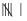6 10–20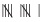11 20–30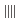4 30–40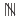5 40–50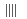4

Question 2: Study the following frequency table and answer the questions given below.

(i) What is the class interval?

(ii) Which class has the lowest frequency?

(iii) Which class has the highest frequency?

(iv) Which two classes have the same frequency?

 Class interval (Daily income in rupees) Frequency (Number of workers) 200–225225–250250–275275–300300–325325–350350–375375–400 25384245172345120 Total 365

(v) What is the lower limit of the class-interval 300–325?

(vi) What is the upper limit of the class interval 275–300?

Solution:

(i) The class interval

= [Upper limit of a class] – [Lower limit of the same class]

= 225 – 200

= 25

(ii) The class 300–325 is having the lowest frequency (which is 17).

(iii) The class 375–400 is having is the heighest frequency (which is 120).

(iv) The class intervals 275–300 and 350–375 have the same frequency (which is 45).

(v) The lower limit of the class interval 300–325 is 300.

(vi) The upper limit of the class interval 275–300 is 300.

Question 3: Observe the following histogram and answer the questions given below: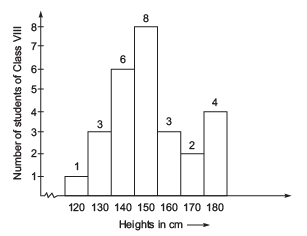(i) What information is being given by the histogram?

(ii) Which group contains the maximum students?

(iii) How many students have a height of 160 cm and more?

(iv) What is the ratio of the number students in 130–140 cm group and the number of students in 160–170 cm groups?

Solution:

(i) This histogram represents heights (in cms) of students of Class VIII.

(ii) The group (150–160) contains maximum number of students (which has 8 students).

(iii) 9 students (3 + 2 + 4) have height of 160 cm or more.

(iv) Number of students in the group of 130 – 140 cm = 3.

Number of students in the group of 160 – 170 cm = 3

∴ The required ratio = 3 : 3 = 1 : 1

Question 4: Look at the adjoining circle graph and answer the following questions.

(i) What is the central angle corresponding to the activities “school and home work”?

(ii) Find the fraction of the circle representing each of these given information.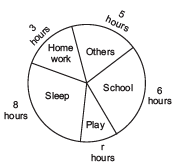Solution:

(i) Since, the total angle at the centre = 360°

i.e. The central angle corresponding to 24 hours = 360°

∴Central angle form school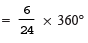= 90°

Central angle for home work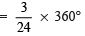= 45°

(ii) Fraction of the circle corresponding to:

Sleeping hours = 8 hours/24 hours = 1/3

Schooling hours = 6 hours/24 hours = 1/4

Playing hours = 2 hours/24 hours = 1/12

Home-work hours = 3 hours/24 hours = 1/8

Other activities hours = 5 hours/24 hours= 5/24

Question 5: The number of students in hostel, speaking different languages is given below. Display the data by a pie chart:

 Language Hindi English Bengali Marathi Tamil Total Number of students 12 40 7 4 9 72

Solution: Central angle of the sector representing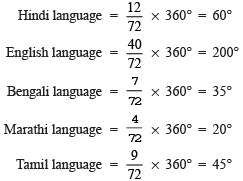Now, the required pie-chart is as given below.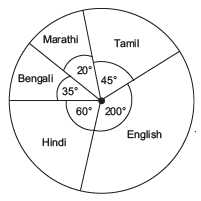Question 6: Numbers 1 to 10 are written on ten separate cards such that one number on one slip. These are mixed well and one slip is chosen from the box without looking into it. What is the probability of:

(i) getting a card on which 7 is written?

(ii) getting a card having two-digit number on it?

(iii) getting a number less than 5?

(iv) getting a number more than 5?

Solution: Total number of out comes = 10

(i) ∵ One card is having 7 on it

∴ Number of favourable outcome = 1

⇒ Probability of getting a card having 7 on it = 1/10

(ii) ∵ Only one card is having two digit number (i.e. 10)

∴ Number of favourable outcome = 1

∴ Probability of getting a card having 10 (two digit number) on it = 1/10

(iii) ∵ The numbers less than 5 are 1, 2, 3, and 4.

∴ Number of favourable outcomes = 4

⇒ Probability of getting a number less then 5 =4/10 or 2/5

(iv) ∵ The numbers more than 5 are 6, 7, 8, 9 and 10.

∴ Number of favourable outcomes = 5

⇒ Probability of getting a number more than 5 = 5/10 or 1/10

Question 7: When a die is thrown, list the outcomes of an event of getting:

(i) A number less than 5.

(ii) A composite number.

(iii) A prime number.

(iv) A number more than 3.

Solution: The number on a die are 1, 2, 3, 4, 5, or 6.

(i) ∵ Numbers less than 5 are 1, 2, 3 and 4.

∴ Possible outcomes = 4

⇒ Probability of getting a number less than 5 =4/6 or 2/3

(ii) ∵ The composite numbers are 4 and 6.

∴ Number of outcomes = 2

⇒ Probability getting a composite number = 2/6 or 1/3

(iii) ∵ The prime numbers are 2, 3 and 5.

∴ Number of outcomes = 3

⇒ Probability of getting a prime number = 3/6 = 1/2

(iv) ∵ Numbers more than 3 are 4, 5, and 6.

∴ Number of outcomes = 3

∴ Probability of getting a number greater than 3 = 3/6 or 1/2

Practice Exercise

Question 1: What is the number of students of Class VIII whose marks obtained in an examination are expressed in the following frequency distribution.

 Marks Tally Marks Frequency 0 – 5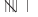6 5–10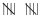10 10–15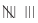8 15–20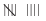9 20–25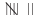7 Total ...

Question 2: The marks scored by 20 students in a test are given below:

84, 57, 53, 89, 41, 57, 47, 64, 58, 44, 53, 72, 51, 78, 71, 62, 56, 68, 54, 42 Complete the following frequency table:

 Marks in class interval Tally marks No. of students 40–5050–6060–7070–8080–90

(i) What is the upper limit of 40–50?

(ii) What is the upper limit of 70–80? (iii) What is the class size?

Answers: (i) 50 (ii) 80 (iii) 10

Question 3: The following pie-chart represents the marks scored by a student. If he obtained 540 as total marks, answer the following questions: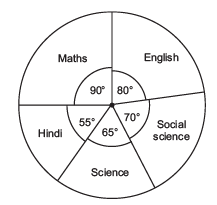(i) In which subject did the student score 120 marks?

(ii) What is the difference in the marks obtained in Maths and English?

(iii) In which subject did he get minimum marks?

Answers: (i) English (ii) 15 (iii) Hindi

Question 4: A die is thrown. What is the probability of getting:

(i) an even number?

(ii) an odd number?

(iii) A number between 3 and 6?

Answers: (i)  1/6 (ii) 2/3 (iii) 1/3

Question 5: What is the probability of a number selected from the numbers 1, 2, 3, …., 20 such that it is a prime number?

Question 6: A bag contains 3 blue and 2 red balls. A ball is drawn at random. What is the probability of drawing a red ball?

Offer running on EduRev: Apply code STAYHOME200 to get INR 200 off on our premium plan EduRev Infinity!

## Mathematics (Maths) Class 8

135 videos|321 docs|48 tests

,

,

,

,

,

,

,

,

,

,

,

,

,

,

,

,

,

,

,

,

,

;